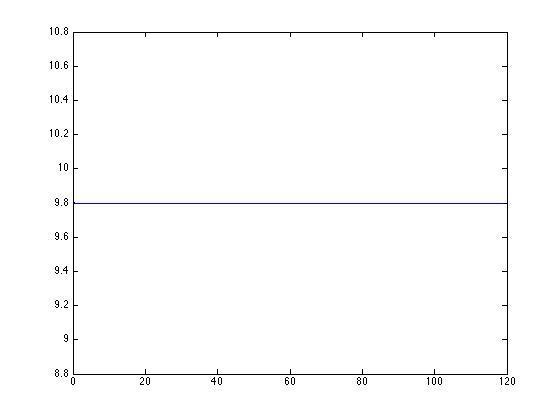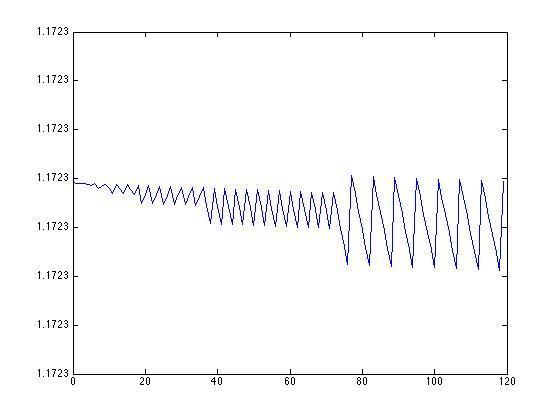# Plotting cosine in matlab looks really funky

• MATLAB
nabeel17
I have to plot a bunch of cosine waves and then add them up but my problem is that each indiviudal wave looks really weird when I plot and not like a cos wave. The program runs and plots the final result but I'm not sure how accurate it is because when I plot each individual cos wave I know it should not look like that

This is my method,

t=[0:119];
a1=9.8;
f1=200;
P1=0;
s1=a1*cos(2*pi*f1*t+P1);

t=[0:119];
a2=7.6;
f2=145;
P2=30;
s2=a2*cos(2*pi*f2*t+P2);

t=[0:119];
a3=5.4;
f3=93;
P3=70;
s3=a3*cos(2*pi*f3*t+P3);

t=[0:119];
a4=3.2;
f4=58;
P4=160;
s4=a4*cos(2*pi*f4*t+P4);

t=[0:119];
a5=1.0;
f5=35;
P5=320;
s5=a5*cos(2*pi*f5*t+P5);

S1=s1+s2+s3+s4+s5;
plot(t,S1)

any help would be appreciated

Homework Helper
You'll need to show us the plots if we are to see what you are talking about.

nabeel17
You'll need to show us the plots if we are to see what you are talking about.These are what s1 and s2 look like. They should both just look like regular cos waves but Im not sure why theyre looking like that. They should just be cosine waves with certain frequencies aplitudes and phase shifts given by a, f, and P.

Last edited:
Mentor
Your range for t is the interval [0, 119]. The cos function in Matlab probably expects its argument to be in radians, not degrees that I believe you think you are using. To convert radians to degrees, multiply the degree value by 180/##\pi##.

Homework Helper
You probably need to define a smaller step in the t vector.

Matlab does not know about the whole curve - what you are doing is sampling the curve at particular points.
I your first curve, you are sampling at intervals that are a whole number of periods - so you get the same value for the sine wave for every value of t. In the second one, the sampling interval means that the data points alternate between low and high values - giving a saw-tooth effect.

For matlab to show you the curve of form ##\sin(2\pi t/T)## you have to set up a time vector t=a:dt:b; so that ##dt<T/10## ... the more data points you get in one period, the smoother the resulting plot will look.

So for the first one - T = 1/f = 1/200, so t=0:1/2048:119;
Even so the graph is going to be very squashed to fit it into a matlab graph window.
Try plotting for shorter lengths of time.

The scale-factor ##2\pi f## in each equation should make sure the argument is already in radians.
JIC: check that your units for frequency are the inverse of your units for time.
i.e. f=200 should give 200 cycles in 1 time unit.

 the P1, P2 etc values should be in radians too.

Last edited:
•1 person
nabeel17
Ahhh that fixed up the graphs. My problem now is that i have 5 cosine functions with different frequencies and amplitudes. They all range from 0:119ms so i wrote it as

t=[0:0.001:0.119] i chose 0.001 for dt because i can only have 120 time steps. The problem with this is that though it gives a nice cosine curve for some of the functions, it does not for all. Is there a way to get around this?

Homework Helper
It could mean you cannot plot the whole 119ms on a single graph.
How come you are restricted to only 120 time steps?

BTW: if you want to have N steps in a range from a to b, then you want: t=a:(b-a)/N:b; .... which will give you N+1 data points. (Notice you don't need the square brackets to set this up?)

--- aside --------------------------

I'd put this at the top of your m-file:
Code:
### Initialization ###
ti=0;              # initial time (ms)
tf=119;            # final time (ms)
N=120;             # data points
dt=(b-a)/(N-1);    # time-step
t=ti:dt:tf;        # time axis
... that means that you can change the range etc of all the graphs by editing just one line at the top of the m-file. Gives you more freedom to play around.

Notice I put time in milliseconds rather than seconds (what you did)?
That means I have to convert the frequencies to cycles per millisecond - which you didn't need to do.

It is good practice if the number of steps is close to a power of 2 rather than 10.
This is because 0.1 is an irrational number in binary - so the computer has to round it off.
Probably won't matter for this calculation, but when you are doing math with lots of iterations, small rounding errors on each step can blow up quite fast.

Last edited:
•1 person
Homework Helper
Should be long enough now that this won't matter...

Setting up the m-file carefully makes it easier to troubleshoot.
You can also combine a lot of calculations into a few lines by exploiting matrix properties.
Notice how all five calculations use the one calculation - you can add more calculations just by editing the vector data, and change the parameters of the calculations by editing the initialization.

Code:
### Initialization ###
ti=0;              # initial time (ms)
tf=119;            # final time (ms)
N=120:             # No. data points
dt=(b-a)/(N-1)     # time-step

### vector data ###
# time is a row, the rest are columns
t=ti:dt:tf;                         # times
A= [9.8, 7.6, 5.4, 3.2, 1.0]';      # amplitudes
f= [145, 93, 58, 35]'./1000;        # frequencies
p= [0, 30, 70, 160, 320]'.*pi/180;  # phases

### main calculation ###
s=diag(A)*cos( f*t + diag(p)*ones( size(f*t) ) );

### display ###

## All graphs on the same axis:
figure(1)
plot(t,s)

## Separate axes, same figure.
figure(2)
for k=1:5
subplot(5,1,k)
plot(t,s(k,:))
end

stop

•1 person
nabeel17
Ahhh, thats very useful, thank you!